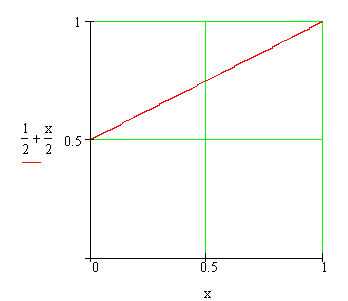Calculus on Demand at Dartmouth College Lecture 22 | Index | Lecture 24 Lecture 23Resources

Math 3 Course Syllabus
Practice Exams

Contents

In this lecture we state and prove the Fundamental Theorem of Calculus. This theorem has that name because it relates derivatives and integrals, the two main branches of calculus. We also discuss some resulting techniques for evaluating definite integrals.

Quick Question

What is the area under the graph of f(x) = 1/2 + x/2 and above the interval [0,1]? Now let F(x) = x/2 + x2/4; what is F(1) − F(0)?Outline

Outlines for
The Fundamental Theorem of Calculus
Techniques of Integration

Textbook

The Fundamental Theorem of Calculus
Techniques of Integration